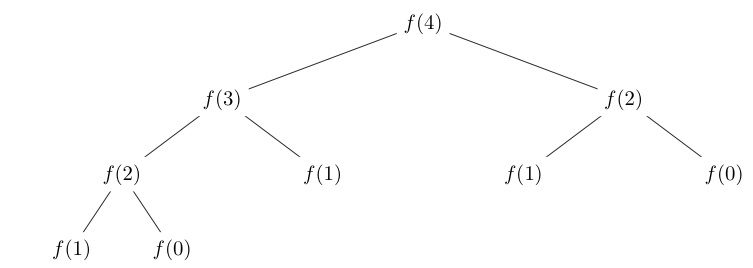# Fibonacci Numbers

suggest change

Fibonacci Numbers are a prime subject for dynamic programming as the traditional recursive approach makes a lot of repeated calculations. In these examples I will be using the base case of `f(0) = f(1) = 1`.

Here is an example recursive tree for `fibonacci(4)`, note the repeated computations:Non-Dynamic Programming `O(2^n)` Runtime Complexity, `O(n)` Stack complexity

``````def fibonacci(n):
if n < 2:
return 1
return fibonacci(n-1) + fibonacci(n-2)``````

This is the most intuitive way to write the problem. At most the stack space will be `O(n)` as you descend the first recursive branch making calls to `fibonacci(n-1)` until you hit the base case `n < 2`.

The `O(2^n)` runtime complexity proof that can be seen here: https://stackoverflow.com/questions/360748/computational-complexity-of-fibonacci-sequence. The main point to note is that the runtime is exponential, which means the runtime for this will double for every subsequent term, `fibonacci(15)` will take twice as long as `fibonacci(14)`.

Memoized `O(n)` Runtime Complexity, `O(n)` Space complexity, `O(n)` Stack complexity

``````memo = []
memo.append(1) # f(1) = 1
memo.append(1) # f(2) = 1

def fibonacci(n):
if len(memo) > n:
return memo[n]

result = fibonacci(n-1) + fibonacci(n-2)
memo.append(result) # f(n) = f(n-1) + f(n-2)
return result``````

With the memoized approach we introduce an array that can be thought of as all the previous function calls. The location `memo[n]` is the result of the function call `fibonacci(n)`. This allows us to trade space complexity of `O(n)` for a `O(n)` runtime as we no longer need to compute duplicate function calls.

Iterative Dynamic Programming `O(n)` Runtime complexity, `O(n)` Space complexity, No recursive stack

``````def fibonacci(n):
memo = [1,1] # f(0) = 1, f(1) = 1

for i in range(2, n+1):
memo.append(memo[i-1] + memo[i-2])

return memo[n]``````

If we break the problem down into it’s core elements you will notice that in order to compute `fibonacci(n)` we need `fibonacci(n-1)` and `fibonacci(n-2)`. Also we can notice that our base case will appear at the end of that recursive tree as seen above.

With this information, it now makes sense to compute the solution backwards, starting at the base cases and working upwards. Now in order to calculate `fibonacci(n)` we first calculate all the fibonacci numbers up to and through `n`.

This main benefit here is that we now have eliminated the recursive stack while keeping the `O(n)` runtime. Unfortunately, we still have an `O(n)` space complexity but that can be changed as well.

Advanced Iterative Dynamic Programming `O(n)` Runtime complexity, `O(1)` Space complexity, No recursive stack

``````def fibonacci(n):
memo = [1,1] # f(1) = 1, f(2) = 1

for i in range (2, n):
memo[i%2] = memo + memo

return memo[n%2]``````

As noted above, the iterative dynamic programming approach starts from the base cases and works to the end result. The key observation to make in order to get to the space complexity to `O(1)` (constant) is the same observation we made for the recursive stack - we only need `fibonacci(n-1)` and `fibonacci(n-2)` to build `fibonacci(n)`. This means that we only need to save the results for `fibonacci(n-1)` and `fibonacci(n-2)` at any point in our iteration.

To store these last 2 results I use an array of size 2 and simply flip which index I am assigning to by using `i % 2` which will alternate like so: `0, 1, 0, 1, 0, 1, ..., i % 2`.

I add both indexes of the array together because we know that addition is commutative (`5 + 6 = 11` and `6 + 5 == 11`). The result is then assigned to the older of the two spots (denoted by `i % 2`). The final result is then stored at the position `n%2`

## Notes

• It is important to note that sometimes it may be best to come up with a iterative memoized solution for functions that perform large calculations repeatedly as you will build up a cache of the answer to the function calls and subsequent calls may be `O(1)` if it has already been computed.